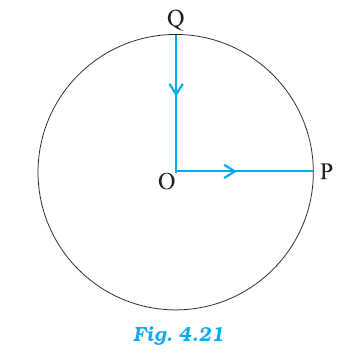## Pages

### Motion in a plane NCERT Solutions Class 11 Physics - Solved Exercise Question 4.9

Question 4.9:

A cyclist starts from the centre O of a circular park of radius 1 km, reaches the edge P of the park, then cycles along the circumference, and returns to the centre along QO as shown in Fig. 4.21. If the round trip takes 10 min, what is the
(a) net displacement,
(b) average velocity, and
(c) average speed of the cyclist ?Solution:

(a) Displacement is given by the minimum distance between the initial and final positions of a body. In the given case, the cyclist comes to the starting point after cycling for 10 minutes. Hence, his net displacement is zero.

(b) Average velocity is given by the relation:
Average velocity = Net Displacement / Total time
Since the net displacement of the cyclist is zero, his average velocity will also be zero.

(c) Average speed of the cyclist is given by the relation:
Average speed = Total path length / Total time
Total path length = OP + PQ + QO
= 1 + (1 (2π X 1) /)  + 1
= 2 + (π / 2)
= 3.570 km
Time taken = 10 min = 10 / 60 = 1 / 6 h
∴ Average speed = 3.570 / (1/6) = 21.42 km/h